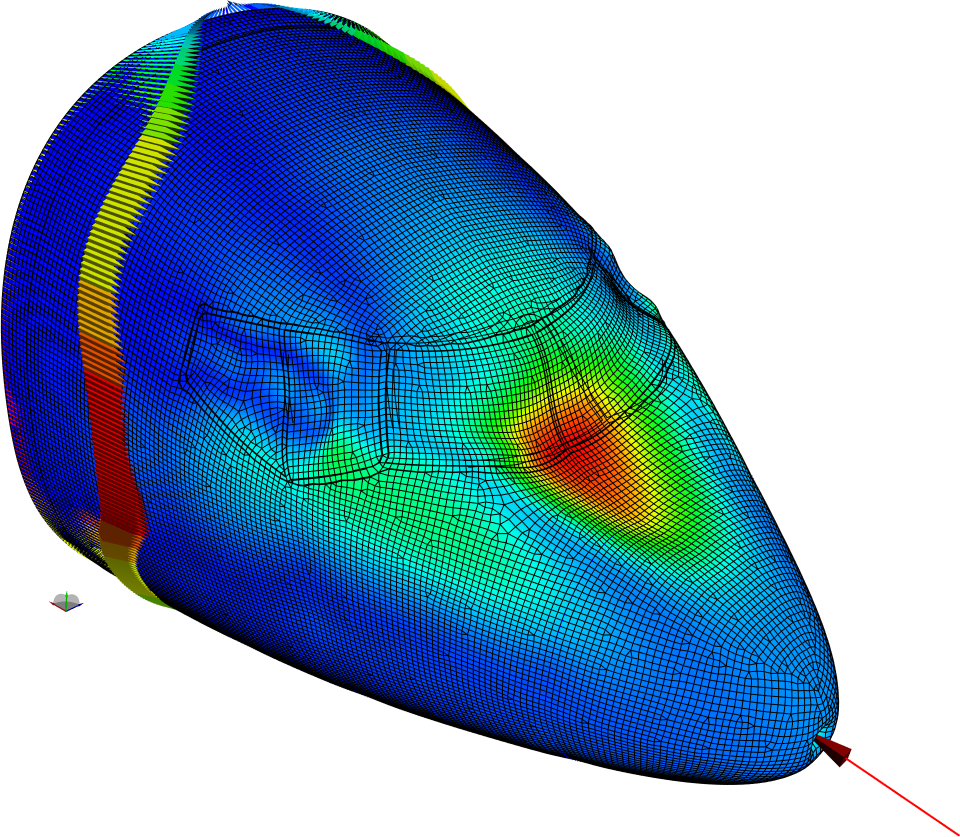# Linear StaticsPERMAS-LS allows for linear elastic calculations, based upon the assumptions of small displacements, small strains and linear material behavior (isotropic or anisotropic). Therefore, this calculation often builds the first step in Finite Element analysis. Subsequent applications are e.g: Contact (CA) or material/-geometrical nonlinearities (NLS). Thorough model checks are being performed to assure the quality (see PERMAS-MQA).
Different kinds of static loadings are available. For free or partially free structures a quasi-static analysis can be performed (Inertia Relief). There, on the basis of a rigid body decoupling the inertia forces are computed which are in equilibrium with the applied forces. Subsequently, a static analysis is performed unter the applied loads and these inertia forces.
• Suppressed degrees of freedom,
• Prescribed degrees of freedom and
• Linear constraints (MPCs).
• Weld connections WLDS.
• Concentrated forces
• Various distributed loads (at elements/nodes or volume based)